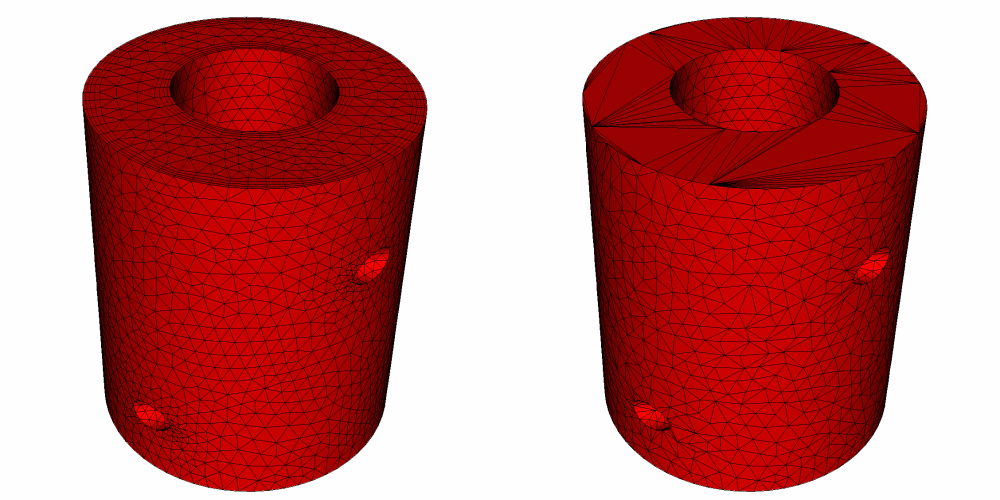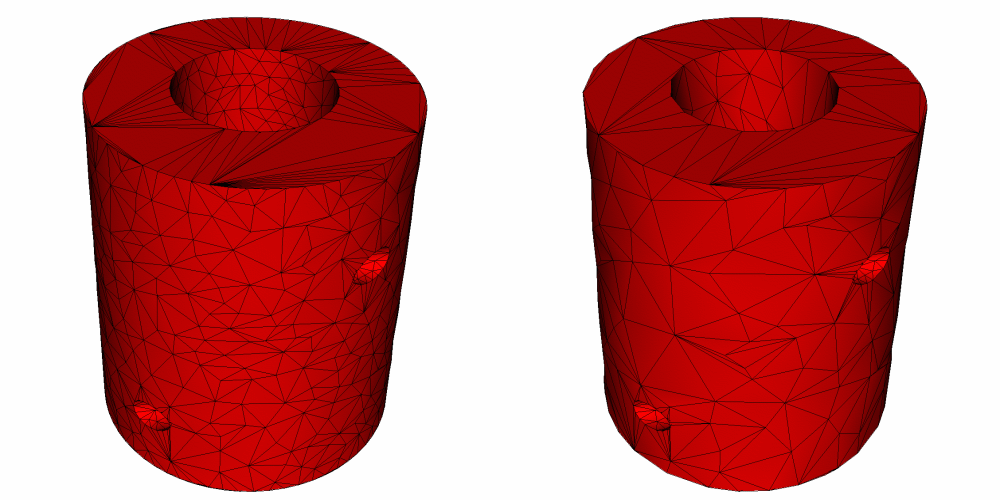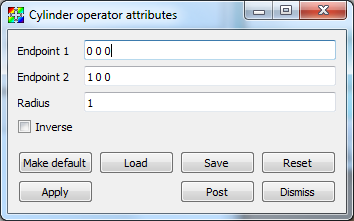# 4.3.7. Decimate operator¶

The Decimate operator, shown in Figure 4.27, removes nodes and cells from an input mesh, reducing the cell count while trying to maintain the overall shape of the original mesh. The Decimate operator can currently operate only on the external surfaces of the input geometry. This means that in order to apply the Decimate operator, you must first apply the ExternalSurface operator, which will be covered later in this chapter. The Decimate operator is not enabled by default but it can be turned on in the Plugin Manager Window.

## 4.3.7.1. Using the Decimate operator¶

The Decimate operator simplifies mesh geometry. This can be useful for producing models that have lower polygon counts than the model before the Decimate operator was applied. Models with lower polygon count can be useful for speeding up operations such as rendering. The Decimate operator has a single knob that influences how many cells are removed from the input mesh. The Target Reduction value is a floating point number in the range (0,1) and it can be set in the Decimate attributes window (see Figure 4.28). The number specified is the proportion of number of polygonal cells in the output dataset “over” the number of polygonal cells in the original dataset. As shown in Figure 4.27, higher values for Target Reduction value cause VisIt to simplify the mesh even more.Fig. 4.27 Decimate operator applied to reduce the number of cells in the mesh. (Left-to-right, top-to-bottom): Original Mesh, Reduction = 0.1, Reduction = 0.5, Reduction = 0.75Fig. 4.28 Decimate attributes window